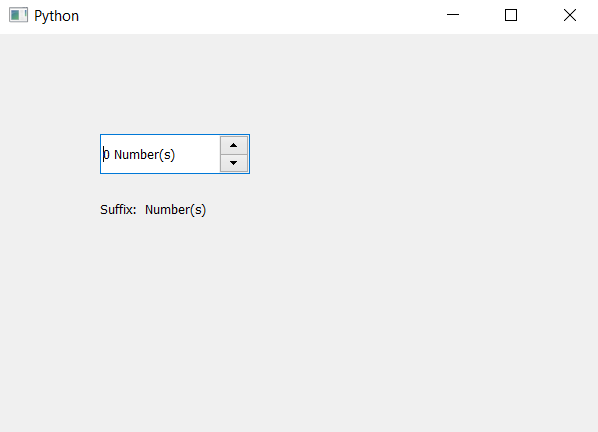Related Articles

# PyQt5 QSpinBox – Accessing Suffix

• Last Updated : 06 May, 2020

In this article we will see how we can access the suffix of the spin box, suffix is the extra text added at the end of the value, it is non editable and permanent. By default there is no suffix set to the spin box although we can set suffix any time with the help of `setSuffix` method.

In order to do this we will use `suffix` method.

Syntax : spin_box.suffix()

Argument : It takes no argument

Return : It returns string

Steps for implementation :
1. Create a spin box
2. Set suffix with the help of setSuffix method
3. Create label to show the suffix
4. Get the suffix with the help of suffix method
5. Show suffix through label

Below is the implementation

 `# importing libraries``from` `PyQt5.QtWidgets ``import` `*` `from` `PyQt5 ``import` `QtCore, QtGui``from` `PyQt5.QtGui ``import` `*` `from` `PyQt5.QtCore ``import` `*` `import` `sys`` ` `class` `Window(QMainWindow):`` ` `    ``def` `__init__(``self``):``        ``super``().__init__()`` ` `        ``# setting title``        ``self``.setWindowTitle(``"Python "``)`` ` `        ``# setting geometry``        ``self``.setGeometry(``100``, ``100``, ``600``, ``400``)`` ` `        ``# calling method``        ``self``.UiComponents()`` ` `        ``# showing all the widgets``        ``self``.show()`` ` `    ``# method for widgets``    ``def` `UiComponents(``self``):`` ` `        ``# creating spin box``        ``self``.spin ``=` `QSpinBox(``self``)`` ` `        ``# setting geometry to spin box``        ``self``.spin.setGeometry(``100``, ``100``, ``150``, ``40``)`` ` `        ``# setting step type``        ``self``.spin.setSuffix(``" Number(s)"``)`` ` `        ``# creating label``        ``label ``=` `QLabel(``self``)`` ` `        ``# setting geometry to the label``        ``label.setGeometry(``100``, ``160``, ``200``, ``30``)`` ` `        ``# getting suffix``        ``suffix ``=` `self``.spin.suffix()`` ` `        ``# setting text to the label``        ``label.setText(``"Suffix: "` `+` `suffix)`` ` `# create pyqt5 app``App ``=` `QApplication(sys.argv)`` ` `# create the instance of our Window``window ``=` `Window()`` ` `# start the app``sys.exit(App.``exec``())`

Output :Attention geek! Strengthen your foundations with the Python Programming Foundation Course and learn the basics.

To begin with, your interview preparations Enhance your Data Structures concepts with the Python DS Course. And to begin with your Machine Learning Journey, join the Machine Learning – Basic Level Course

My Personal Notes arrow_drop_up